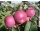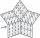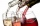# Mini-survey

In the mini-survey of our class about the popularity of individual subjects, it turned out that 11.1% of pupils like mathematics, 18.5% are enjoying languages, 30.4% of pupils like physical education, and the remaining 12 pupils have several popular subjects. How many students are visiting our class?

Result

n =  30

#### Solution:Leave us a comment of example and its solution (i.e. if it is still somewhat unclear...):

Showing 0 comments:Be the first to comment!#### To solve this example are needed these knowledge from mathematics:

Need help calculate sum, simplify or multiply fractions? Try our fraction calculator. Our percentage calculator will help you quickly calculate various typical tasks with percentages. Do you have a linear equation or system of equations and looking for its solution? Or do you have quadratic equation?

## Next similar examples:

1. Jane classWhen asked how many students are in class, Jane said, if we increase the number of students in our class by hundred % and then add half the number of students, we get 100. How many students are in Jane's class?
2. ThereThere are 32 trees of three species in the orchard. 25% cherries, three eighty pears, others plums. How many plums are in the orchard?
3. Profit gainIf 5% more is gained by selling an article for Rs. 350 than by selling it for Rs. 340, the cost of the article is:
4. Three monksThree medieval monks has task to copy 600 pages of the Bible. One rewrites in three days 1 page, second in 2 days 3 pages and a third in 4 days 2 sides. Calculate for how many days and what day the monks will have copied whole Bible when they begin Wednesd
5. Perctentages35% of what number is 35?
6. CompetitorsIn the first round of slalom fell 15% of all competitors and in the second round another 10 racers. Together, 40% of all competitors fell. What was the total number of competitors?
7. Unknown number 23Find 2/3 of unknown number, which is two thirds of the 99.
8. Cinema ticketsCinema sold 180 tickets this Thursday, which is 20%. Monday 14%, Tuesday 6%, Wednesday 9%, Friday 24%, Saturday 12%, and Sunday 15%. How many tickets were sold per week?
9. PupilsThere are 350 girls in the school, and the other 30% of the total number of pupils are boys. How many pupils does the school have?
10. Reducing numberReducing the an unknown number by 28.5% we get number 243.1. Determine unknown number.
11. WineryThe wine processing plant sold 8/15 of its total wine production to the domestic market, 2/7 to the foreign the rest of the wine. How many percents are still in stock?
12. PercentCalculate how many % is the number 26.25 less than the number 105.
13. GirlsThe children's competition was attended by 63 girls, which is 30% of all children's participants. How many children attended this competition?
14. Apples 14Maya bought 18 apples. She used 50% of the apples to make apple pies and 1/3 of the apples was mixed with other fruits to make salad. How many apples were left?
15. Discount saleAt Christmas Sale after a 20% discount cosmetic package was priced at a price of 5 euros and 60 cents; later it went to 3 euros and 80 cents. How much percentage is the total discount?
16. The ballThe ball was discounted by 10 percent and then again by 30 percent. How many percent of the original price is now?
17. Simple equation 5Solve equation with fractions: X × 3/8 = 1/2